Take the Last leap| Aakash BYJU'S JEE (Adv) '23 Mock Test on 28th May Take the Last leap| Aakash BYJU'S JEE (Adv) '23 Mock Test on 28th May

# JEE Main 2022 July 27 – Shift 2 Physics Question Paper with Solutions

The JEE Main 2022 July 27 – Shift 2 Physics Question Paper with Solutions will be available on this page. JEE aspirants can use JEE Main 2022 answer keys to find the correct solutions and also to calculate their scores. Students are required to solve and learn the JEE Main 2022 question paper with solutions so as to boost their performance in the upcoming JEE Main exams. Practising the JEE Main 2022 question papers will help students to improve their confidence and be stress-free during the entrance exam.

## JEE Main 2022 July 27th Shift 2 Physics Question Paper and Solutions

SECTION – A

Multiple Choice Questions: This section contains 20 multiple choice questions. Each question has 4 choices (1), (2), (3) and (4), out of which ONLY ONE is correct.

1. An expression of energy density is given by

$$\begin{array}{l} u=\frac{\alpha}{\beta}\sin\left(\frac{\alpha x}{kt}\right) \end{array}$$
, where α, β are constants, x isdisplacement, k is Boltzmann constant and t is the temperature. The dimensions of β willbe

$$\begin{array}{l}\left(A\right) \left[ML^2T^{–2}\theta^{–1}\right]\\ \left(B\right) \left[M^0L^2T^{–2}\right]\\ \left(C\right) \left[M^0L^0T^0\right]\\ \left(D\right) \left[M^0L^2T^0\right]\end{array}$$

Sol.

$$\begin{array}{l} u=\frac{\alpha}{\beta}\sin\left(\frac{\alpha x}{kt}\right) \end{array}$$
$$\begin{array}{l} \left[\alpha\right]=\left[\frac{kt}{x}\right]=\frac{\left[\text{Energy}\right]}{\left[\text{Distance}\right]}\end{array}$$
$$\begin{array}{l} \left[\beta\right]=\frac{\left[\alpha\right]}{\left[u\right]}\end{array}$$
$$\begin{array}{l} =\frac{\left[\text{Energy}\right]/\left[\text{Distance}\right]}{\left[\text{Energy}\right]/\left[\text{Volume}\right]}= \left[L^2\right] \end{array}$$

2. A body of mass 10 kg is projected at an angle of 45° with the horizontal. The trajectory of the body is observed to pass through a point (20, 10). If T is the time of flight, then its momentum vector, at time t = T/√2, is _________.

$$\begin{array}{l}\left[\text{Take}~ g = 10 \text{m/s}^2\right]\end{array}$$

$$\begin{array}{l} (\text{A})\ 100\hat{i}+\left(100\sqrt{2}-200\right)\hat{j}\end{array}$$
$$\begin{array}{l} (\text{B})\ 100\sqrt{2}\hat{i}+\left(100-200\sqrt{2}\right)\hat{j}\end{array}$$
$$\begin{array}{l} (\text{C})\ 100\hat{i}+\left(100-200\sqrt{2}\right)\hat{j}\end{array}$$
$$\begin{array}{l} (\text{D})\ 100\sqrt{2}\hat{i}+\left(100\sqrt{2}-200\right)\hat{j}\end{array}$$

Sol. m = 10 kg

$$\begin{array}{l}\theta = 45^\circ\end{array}$$
$$\begin{array}{l} y=x\tan\theta\left(1-\frac{x}{R}\right) \end{array}$$
$$\begin{array}{l} \Rightarrow 10=20\left(1-\frac{20}{R}\right)\\\Rightarrow R = 40\end{array}$$
$$\begin{array}{l} 40=\frac{u^2}{10}\Rightarrow u=20\end{array}$$
$$\begin{array}{l} \Rightarrow T=\frac{2\times 20\times \frac{1}{\sqrt{2}}}{10}=\frac{4}{\sqrt{2}}\text{ s}\Rightarrow t = 2\text{ s} \end{array}$$
$$\begin{array}{l} at~ t = 2,\overrightarrow{v}=\left(10\sqrt{2}\hat{i}\right)+\left(10\sqrt{2}-2\times 10\right)\hat{j}\end{array}$$
$$\begin{array}{l} \Rightarrow \overrightarrow{p} =10\left[10\sqrt{2}\hat{i}+\left(10\sqrt{2}-20\right)\hat{j}\right]\end{array}$$
$$\begin{array}{l} =100\sqrt{2}\hat{i}+\left(100\sqrt{2}-200\right)\hat{j} \end{array}$$

3. A block of mass M slides down on a rough inclined plane with constant velocity. The angle made by the inclined plane with horizontal is θ. The magnitude of the contact force will be

$$\begin{array}{l} (\text{A})\ Mg \end{array}$$
$$\begin{array}{l} (\text{B})\ Mg cos \theta\end{array}$$
$$\begin{array}{l} (\text{C})\ \sqrt{Mg\sin\theta+Mg\cos\theta} \end{array}$$
$$\begin{array}{l} (\text{D})\ Mg\sin\theta\sqrt{1+\mu}\end{array}$$

Sol. As the body is moving with constant velocity so forces acting on the body must be balanced.

⇒ Contact force from incline should balance weight of the body.

$$\begin{array}{l}\Rightarrow \left|F_{contact}\right| = Mg\end{array}$$

4. A block ‘A’ takes 2 s to slide down a frictionless incline of 30° and length ‘l’, kept inside a lift going up with uniform velocity ‘v’. If the incline is changed to 45°, the time taken by the block, to slide down the incline, will be approximately

(A) 2.66 s

(B) 0.83 s

(C) 1.68 s

(D) 0.70 s

Sol.

$$\begin{array}{l}\theta_1 = 30 ^\circ, \theta_2 = 45^\circ\end{array}$$
$$\begin{array}{l}a_1 = gsin \theta_1 = 5 m/s^2, a_2 = gsin \theta_2\end{array}$$
$$\begin{array}{l} =5\sqrt{2}\text{ m/s}^2\end{array}$$
$$\begin{array}{l} \frac{t_1}{t_2}=\frac{\sqrt{\frac{2l}{a_1}}}{\sqrt{\frac{2l}{a_2}}}=\sqrt{\frac{a_2}{a_1}} \end{array}$$
$$\begin{array}{l}\frac{t_1}{t_2}=\left(2\right)^{1/4}\\ t_2 = (2)^{3/4}\\ \approx 1.68 ~\text{s}\end{array}$$

5. The velocity of the bullet becomes one third after it penetrates 4 cm in a wooden block. Assuming that bullet is facing a constant resistance during its motion in the block. The bullet stops completely after travelling at (4 + x) cm inside the block. The value of x is

(A) 2.0

(B) 1.0

(C) 0.5

(D) 1.5

Sol. S = 4 cm

$$\begin{array}{l} v_4’=\frac{v}{3}, a = \text{constant}\\v_{4+x} = 0\end{array}$$
$$\begin{array}{l} \left(v^2-\frac{v^2}{a}\right)=2a\left(4\right) \end{array}$$
$$\begin{array}{l}\left(v^2 – 0\right) = 2a\left(4 + x\right)\end{array}$$
$$\begin{array}{l} \frac{4}{4+x}=\frac{8}{9}\end{array}$$
$$\begin{array}{l}\Rightarrow x = 0.5 \text{m}\end{array}$$

6. A body of mass m is projected with velocity λve in vertically upward direction from the surface of the earth into space. It is given that ve is escape velocity and λ< 1. If air resistance is considered to be negligible, then the maximum height from the centre of earth, to which the body can go, will be:

$$\begin{array}{l} (\text{A})\ \frac{R}{1+\lambda^2} \end{array}$$
$$\begin{array}{l} (\text{B})\ \frac{R}{1-\lambda^2} \end{array}$$
$$\begin{array}{l} (\text{C})\ \frac{R}{1-\lambda} \end{array}$$
$$\begin{array}{l} (\text{D})\ \frac{\lambda^2R}{1-\lambda^2} \end{array}$$

Sol. Using energy conservation

$$\begin{array}{l} -\frac{GM_em}{R_e}+\frac{1}{2}m\left(\lambda\sqrt{\frac{2GM_e}{R_e}}\right)^2=-\frac{GM_em}{r}\end{array}$$
$$\begin{array}{l} \frac{GM_em}{r}=\frac{GM_em}{R_e}-\frac{GM_em}{R_e}\lambda^2\end{array}$$
$$\begin{array}{l} r=\frac{R_e}{1-\lambda^2}\end{array}$$

7. A steel wire of length 3.2 m (Ys = 2.0 × 1011 Nm-2) and a copper wire of length 4.4 m (Yc = 1.1 × 1011 Nm-2), both of radius 1.4 mm are connected end to end. When stretched by a load, the net elongation is found to be 1.4 mm. The load applied, in Newton, will be:

$$\begin{array}{l} \left(\text{Given } \pi = \frac{22}{7}\right) \end{array}$$

(A) 360

(B) 180

(C) 1080

(D) 154

Sol.

$$\begin{array}{l}\triangle l_s + \triangle l_c = 1.4\end{array}$$
$$\begin{array}{l} \frac{Wl_s}{Y_sA}+\frac{Wl_c}{Y_c\times A}=1.4\times 10^{-3}\end{array}$$
$$\begin{array}{l} W=\frac{1.4\times 10^{-3}}{\left[\frac{3.2}{2\times\left(\pi\times1.4\times10^{-3}\right)^2}+\frac{4.4}{1.1\times\left(\pi\times1.4\times10^{-3}\right)^2}\right]\frac{1}{10^{+11}}} \end{array}$$
$$\begin{array}{l} W\simeq154\text{ N} \end{array}$$

8. In 1st case, Carnot engine operates between temperatures 300 K and 100 K. In 2nd case, as shown in the figure, a combination of two engines is used. The efficiency of this combination (in 2nd case) will be: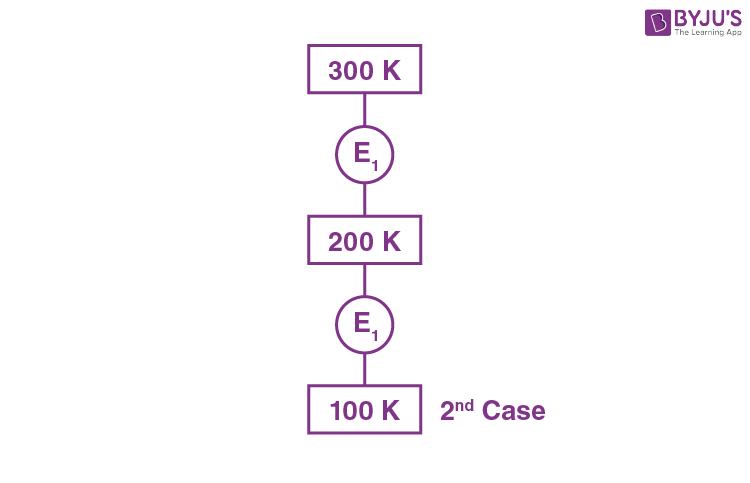(A) Same as the 1st case

(B) Always greater than the 1st case

(C) Always less than the 1st case

(D) May increase or decrease with respect to the 1st case

Sol.

$$\begin{array}{l} \eta_\text{net}=\frac{W_1+W_2}{Q_1}\end{array}$$
$$\begin{array}{l} \eta_\text{net}=\frac{W_1}{Q_1}+\frac{W_2}{Q_1}\end{array}$$
$$\begin{array}{l} \eta_\text{net}=\eta_1+\frac{W_2}{Q_2}\times\frac{Q_2}{Q_1}\end{array}$$
$$\begin{array}{l}\eta_{net} = \eta_1 + \left(\eta_2\right)\left(1 – \eta_1 \right)\\ 1 – \eta_1 < 1\\ \Rightarrow \eta_{net}<\eta_1 + \eta_2\end{array}$$

9. Which statements are correct about degrees of freedom?

(A) A molecule with n degrees of freedom has n2 different ways of storing energy.

(B) Each degree of freedom is associated with (1/2)RT average energy per mole.

(C) A monatomic gas molecule has 1 rotational degree of freedom whereas diatomic molecule has 2 rotational degrees of freedom.

(D) CH4 has a total of 6 degrees of freedom.

Choose the correct answer from the option given below:

(A) (B) and (C) only

(B) (B) and (D) only

(C) (A) and (B) only

(D) (C) and (D) only

Sol. Statement A is incorrect, statement B is correct by equipartition of energy. Statement C is incorrect as monoatomic does not have any rotational degree of freedom and CH4 is a polyatomic gas so it has 6 degree of freedom. So only B and D are correct.

10. A charge of 4 μC is to be divided into two. The distance between the two divided charges is constant. The magnitude of the divided charges so that the force between them is maximum, will be:

(A) 1 μC and 3 μC

(B) 2 μC and 2 μC

(C) 0 and 4 μC

(D) 1.5 μC and 2.5 μC

Sol.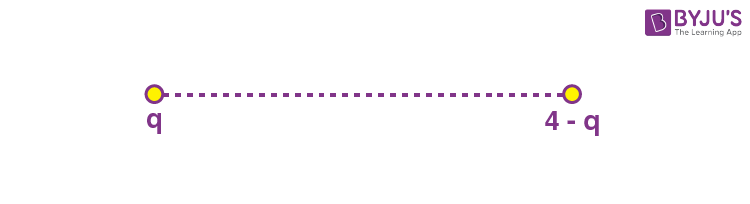so

$$\begin{array}{l} F=\frac{kq\left(4-q\right)\times10^{-12}}{r^2} \end{array}$$

so Fmax will be at

$$\begin{array}{l}q = 2~\mu \text{C}\end{array}$$

11. (A) The drift velocity of electrons decreases with the increase in the temperature of conductor.

(B) The drift velocity is inversely proportional to the area of cross-section of given conductor.

(C) The drift velocity does not depend on the applied potential difference to the conductor.

(D) The drift velocity of electron is inversely proportional to the length of the conductor.

(E) The drift velocity increases with the increase in the temperature of conductor.

Choose the correct answer from the options given below

(A) (A) and (B) only

(B) (A) and (D) only

(C) (B) and (E) only

(D) (B) and (C) only

Sol. Vd decreases with increase in temperature and Vd is inversely proportional to the area of cross section.

12. A compass needle of oscillation magnetometer oscillates 20 times per minute at a place P of dip 30°. The number of oscillations per minute become 10 at another place Q of 60° dip. The ratio of the total magnetic field at the two places (BQ: BP) is

$$\begin{array}{l} (\text{A})\ \sqrt{3}:4 \end{array}$$
$$\begin{array}{l} (\text{B})\ 4:\sqrt{3} \end{array}$$
$$\begin{array}{l} (\text{C})\ \sqrt{3} :2\end{array}$$
$$\begin{array}{l} (\text{D})\ 2:\sqrt{3}\end{array}$$

Sol.

$$\begin{array}{l} T\propto \frac{1}{\sqrt{B\cos\delta}}\end{array}$$
$$\begin{array}{l} \Rightarrow\ \frac{T_1}{T_2}=\sqrt{\frac{B_2\cos\delta_2}{B_1\cos\delta_1}}\end{array}$$
$$\begin{array}{l} \Rightarrow\ \frac{3\text{ s}}{6\text{ s}}=\sqrt{\frac{B_2}{B_1}\times\frac{\frac{1}{2}}{\frac{\sqrt{3}}{2}}}\end{array}$$
$$\begin{array}{l} \Rightarrow\ \frac{B_2}{B_1}=\left(\frac{1}{2}\right)^2\times\sqrt{3}=\frac{\sqrt{3}}{4}\end{array}$$

13. A cyclotron is used to accelerate protons. If the operating magnetic field is 1.0 T and the radius of the cyclotron ‘dees’ is 60 cm, the kinetic energy of the accelerated protons in MeV will be

$$\begin{array}{l}\left[\text{Use}~ m_p = 1.6 \times 10^{-27}~\text{kg} , e = 1.6 \times 10^{-19}~ \text{C}\right]\end{array}$$

(A) 12

(B) 18

(C) 16

(D) 32

Sol.

$$\begin{array}{l} R=\frac{mv}{Bq}=\frac{\sqrt{2mk}}{Bq}\end{array}$$
$$\begin{array}{l} \Rightarrow\ K=\frac{B^2q^2R^2}{2m}\end{array}$$
$$\begin{array}{l} =\frac{\left(1.6\times10^{-19}\right)^2\times0.6^2}{2\times1.6\times10^{-27}}\text{J} = 18\ \text{MeV}\end{array}$$

14. A series LCR circuit has

$$\begin{array}{l}L = 0.01 \text{H}, R = 10~\Omega~\text{and}~C = 1 \mu\text{F}\end{array}$$
and it is connected to ac voltage of amplitude (Vm)50 V. At frequency 60% lower than resonant frequency, the amplitude of current will be approximately

(A) 466 mA

(B) 312 mA

(C) 238 mA

(D) 196 mA

Sol. ω = 0.4 ω0 ….(i)

$$\begin{array}{l} \Rightarrow I=\frac{V}{Z}=\frac{50}{\sqrt{R^2+\left(\omega L-\frac{1}{\omega C}\right)^2}}\cdots\left(ii\right)\end{array}$$
$$\begin{array}{l}\Rightarrow I = 238\ \text{mA}\end{array}$$

15. Identify the correct statements from the following descriptions of various properties of electromagnetic waves.

(A) In a plane electromagnetic wave electric field and magnetic field must be perpendicular to each other and direction of propagation of wave should be along electric field or magnetic field.

(B) The energy in electromagnetic wave is divided equally between electric and magnetic fields.

(C) Both electric field and magnetic field are parallel to each other and perpendicular to the direction of propagation of wave.

(D) The electric field, magnetic field and direction of propagation of wave must be perpendicular to each other.

(E) The ratio of amplitude of magnetic field to the amplitude of electric field is equal to speed of light.

Choose the most appropriate answer from the options given below

(A) (D) only

(B) (B) & (D) only

(C) (B), (C) & (E) only

(D) (A), (B) & (E) only

Sol. In an EM wave :

1. $$\begin{array}{l} \overrightarrow{E}\perp \overrightarrow{B} \end{array}$$
2. $$\begin{array}{l} \overrightarrow{V}\equiv \overrightarrow{E}\times \overrightarrow{B} \end{array}$$
3. Energy is equally divided
4. $$\begin{array}{l} \left|\overrightarrow{V}\right|=\left|\overrightarrow{E}\right|I\left|\overrightarrow{B}\right|\end{array}$$

16. Two coherent sources of light interfere. The intensity ratio of two sources is 1 : 4. For this interference pattern if the value of

$$\begin{array}{l} \frac{I_\text{max}+I_\text{min}}{I_\text{max}-I_\text{min}}\ \text{is equal to}\ \frac{2\alpha+1}{\beta+3},\end{array}$$
then α/β will be

(A) 1.5

(B) 2

(C) 0.5

(D) 1

Sol.

$$\begin{array}{l} I_\text{max}=\left(\sqrt{I_1}+\sqrt{I_2}\right)^2\end{array}$$
$$\begin{array}{l} I_\text{min}=\left(\sqrt{I_1}-\sqrt{I_2}\right)^2\end{array}$$
$$\begin{array}{l} \therefore\ \frac{I_\text{max}+I_\text{min}}{I_\text{max}-I_\text{min}}=\frac{2\left(I_1+I_2\right)}{4\times\sqrt{I_1I_2}}\end{array}$$
$$\begin{array}{l} =\frac{1}{2}\times\frac{\left(\frac{I_1}{I_2}+1\right)}{\sqrt{\frac{I_1}{I_2}}}\end{array}$$
$$\begin{array}{l} =\frac{1}{2}\times\frac{\left(\frac{1}{4}+1\right)}{\left(\frac{1}{2}\right)}\end{array}$$
$$\begin{array}{l} =\frac{5}{4}=\frac{2\times2+1}{1+3} \end{array}$$
$$\begin{array}{l} \therefore\ \frac{\alpha}{\beta}=\frac{2}{1}=2 \end{array}$$

17. With reference to the observations in photo-electric effect, identify the correct statements from below:

(A) The square of maximum velocity of photoelectrons varies linearly with frequency of incident light.

(B) The value of saturation current increases on moving the source of light away from the metal surface.

(C) The maximum kinetic energy of photo-electrons decreases on decreasing the power of LED (Light emitting diode) source of light.

(D) The immediate emission of photo-electrons out of metal surface can not be explained by particle nature of light/electromagnetic waves.

(E) Existence of threshold wavelength can not be explained by wave nature of light/electromagnetic waves.

Choose the correct answer from the options given below.

(A) (A) & (B) only

(B) (A) & (E) only

(C) (C) & (E) only

(D) (D) & (E) only

Sol.

$$\begin{array}{l} \because\ \frac{1}{2}mv_m^2=h\nu-\phi\end{array}$$
$$\begin{array}{l} \Rightarrow v_m^2\end{array}$$
varies linearly with frequency.

And, threshold wavelength can be explained by particle nature of light.

18. The activity of a radioactive material is 6.4 × 10-4 curie. Its half life is 5 days. The activity will become 5 × 10-6 curie after

(A) 7 days

(B) 15 days

(C) 25 days

(D) 35 days

Sol.

$$\begin{array}{l} \because\ A=\frac{A_0}{2^{\frac{t}{T_{1/2}}}}\end{array}$$
$$\begin{array}{l} \Rightarrow 2^{t/5}=\frac{6.4\times10^{-4}}{5\times10^{-6}}=128=2^7 \end{array}$$
$$\begin{array}{l} \Rightarrow \frac{t}{5}=7 \\\Rightarrow t = 35~\text{days}\end{array}$$

19. For a constant collector-emitter voltage of 8 V, the collector current of a transistor reached to the value of 6 mA from 4 mA, whereas base current changed from 20 μA to 25 μA value. If transistor is in active state, small signal current gain (current amplification factor) will be

(A) 240

(B) 400

(C) 0.0025

(D) 200

Sol.

$$\begin{array}{l} \beta = \frac{\Delta I_C}{\Delta I_B} \end{array}$$
$$\begin{array}{l} =\frac{\left(6-4\right)\times 10^{-3}}{\left(25-20\right)\times10^{-6}}\end{array}$$
$$\begin{array}{l} =\frac{2}{5}\times10^3\\= 400 \end{array}$$

20. A square wave of the modulating signal is shown in the figure. The carrier wave is given by C(t) = 5 sin(8πt) Volt. The modulation index is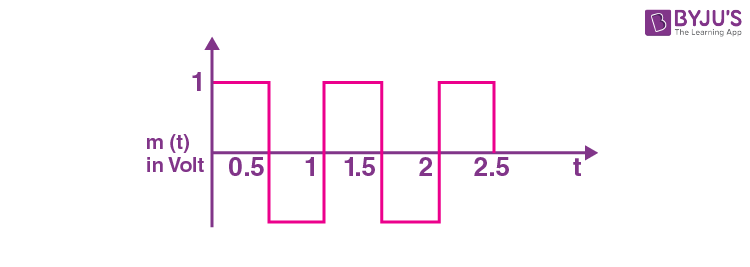(A) 0.2

(B) 0.1

(C) 0.3

(D) 0.4

Sol.

$$\begin{array}{l} \mu=\frac{A_m}{A_c} \end{array}$$
$$\begin{array}{l} =\frac{1}{5}\\= 0.2 \end{array}$$

SECTION – B

Numerical Value Type Questions: This section contains 10 questions. In Section B, attempt any five questions out of 10. The answer to each question is a NUMERICAL VALUE. For each question, enter the correct numerical value (in decimal notation, truncated/rounded-off to the second decimal place; e.g. 06.25, 07.00, –00.33, –00.30, 30.27, –27.30) using the mouse and the on-screen virtual numeric keypad in the place designated to enter the answer.

1. In an experiment to determine the Young’s modulus, steel wires of five different lengths (1, 2, 3, 4 and 5 m) but of same cross section (2 mm2) were taken and curves between extension and load were obtained. The slope (extension/load) of the curves were plotted with the wire length and the following graph is obtained. If the Young’s modulus of given steel wires is x × 1011 Nm–2, then the value of x is ______.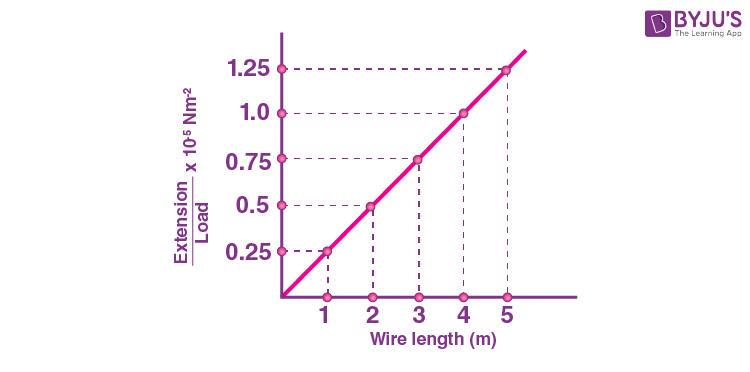Sol.

$$\begin{array}{l} Y=\frac{F\times l}{A\times\Delta l} \end{array}$$
$$\begin{array}{l} =\frac{1}{A}\times \frac{\text{Wire length}}{\frac{\text{Extension}}{\text{load}}}\end{array}$$
$$\begin{array}{l} Y=\frac{1}{A}\times\left(\frac{1}{0.25\times10^{-5}}\right)\\ Y = 10^{11} \times 2\\ \Rightarrow x = 2\end{array}$$

2. In the given figure of meter of bridge experiment, the balancing length AC corresponding to null deflection of the galvanometer is 40 cm. The balancing length, if the radius of the wire AB is doubled, will be _______ cm.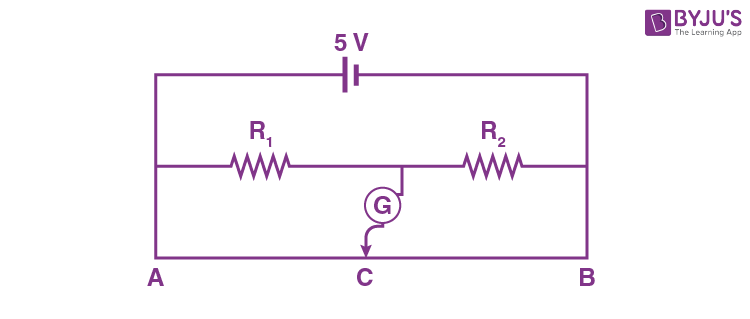Sol. Even if the radius of wire is doubled, the balancing point would not change as

$$\begin{array}{l} \frac{x}{l-x}=\frac{R_1}{R_2}\end{array}$$
, which is not including a term of area.

3. A thin prism of angle 6° and refractive index for yellow light (nY)1.5 is combined with another prism of angle 5° and nY = 1.55. The combination produces no dispersion. The net average deviation (δ) produced by the combination is (1/x)°. The value of x is __________.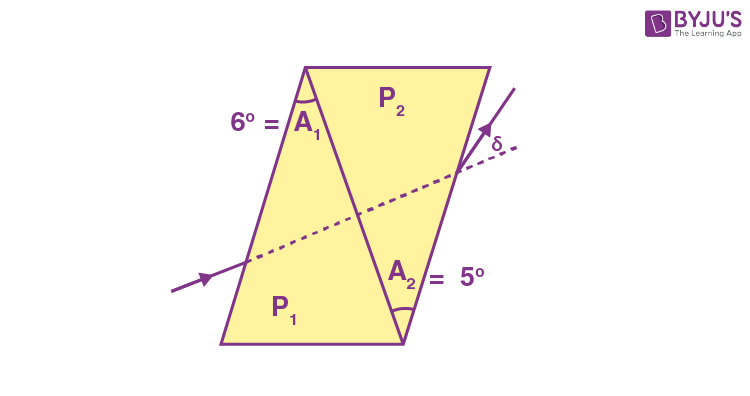Sol.

$$\begin{array}{l}\delta_{net} = \delta_1 + \delta_2 = \left|\left(\mu_1 – 1\right)A_1 – \left(\mu_2 – 1\right)A_2\right|\end{array}$$
$$\begin{array}{l}= \left|3^\circ – 2.75^\circ \right|\\ \delta_\text{net}=\frac{1^\circ}{4}\\ \Rightarrow x = 4\end{array}$$

4. A conducting circular loop is placed in X -Y plane in presence of magnetic field

$$\begin{array}{l} \overrightarrow{B}=\left(3t^3\hat{j}+3t^2\hat{k}\right)\end{array}$$
in SI unit. If the radius of the loop is 1 m, the induced emf in the loop, at time t = 2 s is nπV. The value of n is ______.

Sol.

$$\begin{array}{l}B_\perp = 3t^2\end{array}$$
$$\begin{array}{l} \frac{dB_\perp}{dt}=6t=12 ~at ~t = 2 \end{array}$$
$$\begin{array}{l} \frac{d\phi_1}{dt}=12\times \pi\left(1\right)^2=12\pi\end{array}$$

5. As show in the figure, in the steady state, the charge stored in the capacitor is _________ × 10–6 C.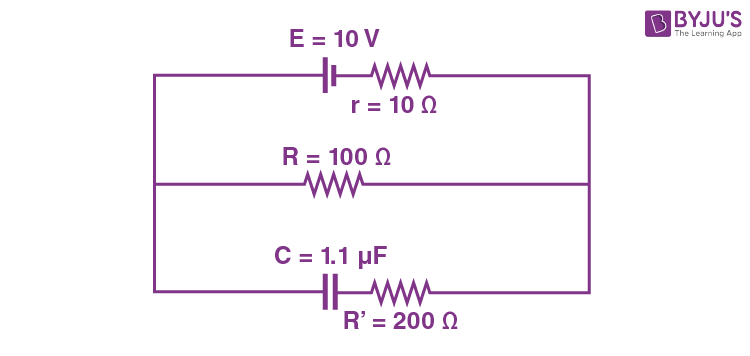Sol. At steady state potential difference across capacitor

$$\begin{array}{l} V_c=\frac{10\times100}{110}\text{V}\end{array}$$

Q = CVc

$$\begin{array}{l} =\frac{1.1\times10^{-6}\times10\times100}{110}\text{C}=10\ \mu \text{C} \end{array}$$

6. A parallel plate capacitor with width 4 cm, length 8 cm and separation between the plates of 4 mm is connected to a battery of 20 V. A dielectric slab of dielectric constant 5 having length 1 cm, width 4 cm and thickness 4 mm is inserted between the plates of parallel plate capacitor. The electrostatic energy of this system will be _______ ε0 J. (Where ε0 is the permittivity of free space)

Sol.

$$\begin{array}{l} d_1= 4 \times 10^{–3}\\ A_1 = 8 \times 4 \times 10^{–4} m^2\\ V = 20 \text{V}\end{array}$$

$$\begin{array}{l} d_2= 4 \times 10^{–3},\\ A_2 = 4 \times 1 \times 10^{–4} \text{m}^2\end{array}$$

$$\begin{array}{l} C_{eq}=\frac{\left(A_1+5A_2-A_2\right)\varepsilon_0}{d}=\frac{3\left(16\right)\times10^{-4}}{4\times10^{-3}}\varepsilon_0\end{array}$$
$$\begin{array}{l} \varepsilon =\frac{1}{2}C_{eq}V^2=\frac{3}{2}\left(\frac{4}{10}\right)\left(400\right)\varepsilon_0=240\varepsilon_0 \end{array}$$

7. A wire of length 30 cm, stretched between rigid supports, has it’s nth and (n + 1)th harmonics at
400 Hz and 450 Hz, respectively. If tension in the string is 2700 N, its linear mass density is _____ kg/m.

Sol.

$$\begin{array}{l} \frac{v}{2l}=50\text{ Hz}\end{array}$$
$$\begin{array}{l} \Rightarrow T=\left[100\times \left(\frac{30}{100}\right)\right]^2\times \mu \end{array}$$
$$\begin{array}{l} \Rightarrow \mu=\frac{2700}{900}=3\end{array}$$

8. A spherical soap bubble of radius 3 cm is formed inside another spherical soap bubble of radius 6 cm. If the internal pressure of the smaller bubble of radius 3 cm in the above system is equal to the internal pressure of the another single soap bubble of radius r cm. The value of r is ___

Sol.

$$\begin{array}{l} \frac{4T}{R_1}+\frac{4T}{R_2}=\frac{4T}{r}\end{array}$$
$$\begin{array}{l} \Rightarrow \frac{1}{r}=\frac{1}{3}+\frac{1}{6}\Rightarrow r= 2\text{ cm}\end{array}$$

9. A solid cylinder length is suspended symmetrically through two massless strings, as shown in the figure. The distance from the initial rest position, the cylinder should be unbinding the strings to achieve a speed of 4 m/s, is ______ cm. (Take g = 10 m/s2).Sol.

$$\begin{array}{l} \alpha = \frac{\left(mg\right)\left(r\right)}{\frac{3}{2}mr^2}=\frac{2g}{3r} \end{array}$$
$$\begin{array}{l} \Rightarrow a=\frac{2g}{3} \end{array}$$
$$\begin{array}{l}\Rightarrow v^2 = 2as\\ 16=\frac{40}{3}\times s\Rightarrow s=0.3\times 4= 120~\text{cm}\end{array}$$

10. Two inclined planes are placed as shown in figure. A block is projected from the point A of inclined plane AB along its surface with a velocity just sufficient to carry it to the top point B at a height
10 m. After reaching the point B the block sides down on inclined plane BC. Time it takes to reach to the point C from point A is t(√2 + 1) s. The value of t is ______. (Use g = 10 m/s2)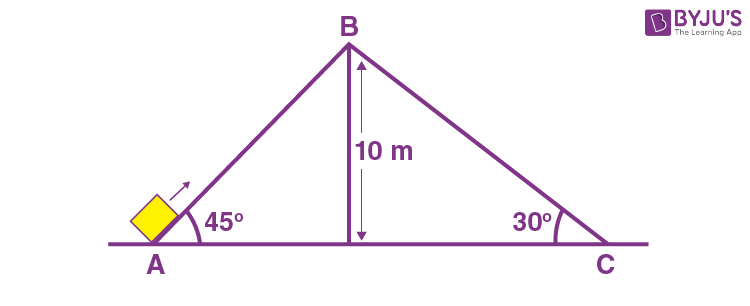Sol.

$$\begin{array}{l} AB=10\sqrt{2}\text{ m} \end{array}$$
$$\begin{array}{l} v_A=\sqrt{2\times 10\times10}=10\sqrt{2}\text{ m/s}\end{array}$$
$$\begin{array}{l} v_C=10\sqrt{2}\text{ m/s}\end{array}$$
$$\begin{array}{l} a_{BC}=g\sin\left(30^\circ\right)=5\text{ m/s}^2 \end{array}$$
$$\begin{array}{l} t_{BC}=2\sqrt{2}\text{ s}\left(\frac{v_c}{a_{BC}}\right) \end{array}$$
$$\begin{array}{l} t_{AB}=\frac{v_A}{5\sqrt{2}}=2\text{ s} \end{array}$$
$$\begin{array}{l}t_{AB}+t_{BC}=2\left(\sqrt{2}+1\right)\\\Rightarrow t=2\end{array}$$

### Download PDF of JEE Main 2022 July 27 Shift 2 Physics Paper & Solutions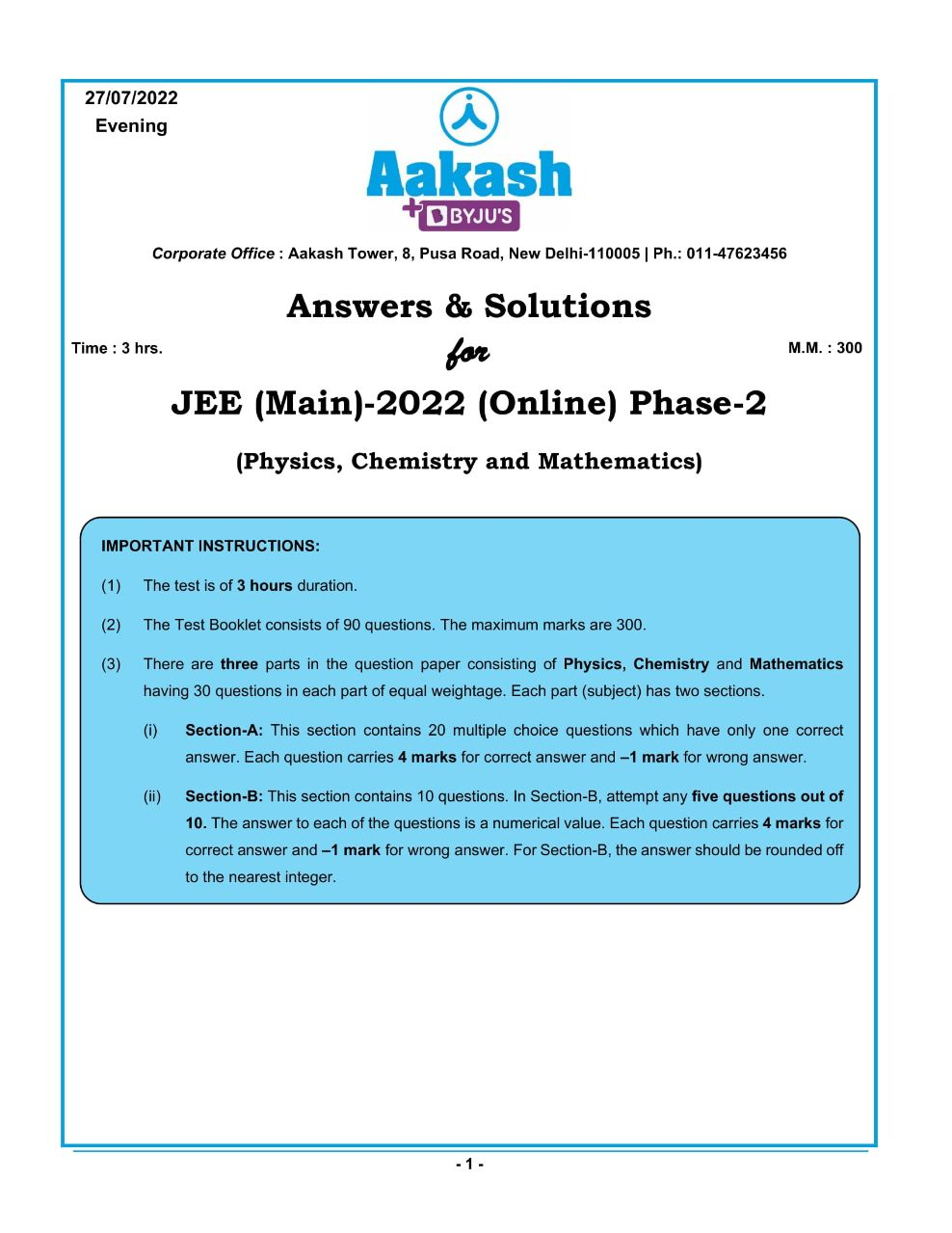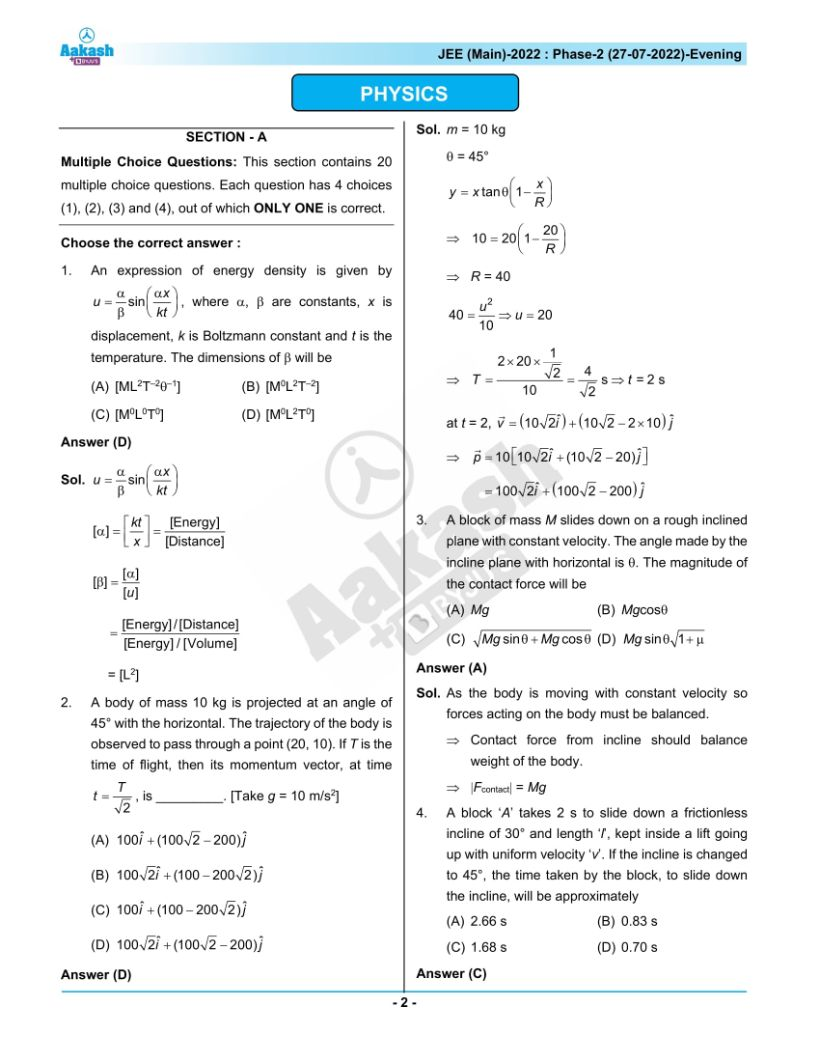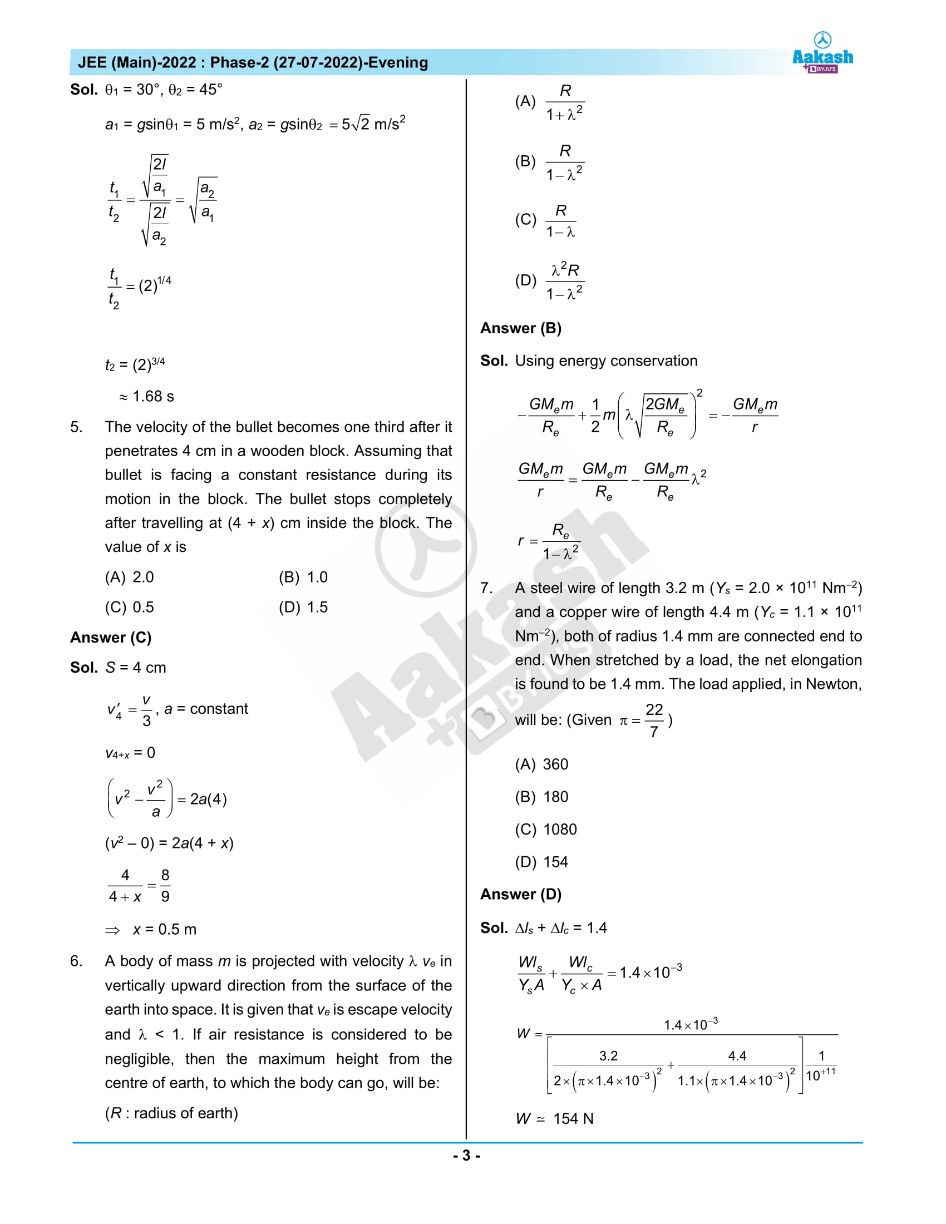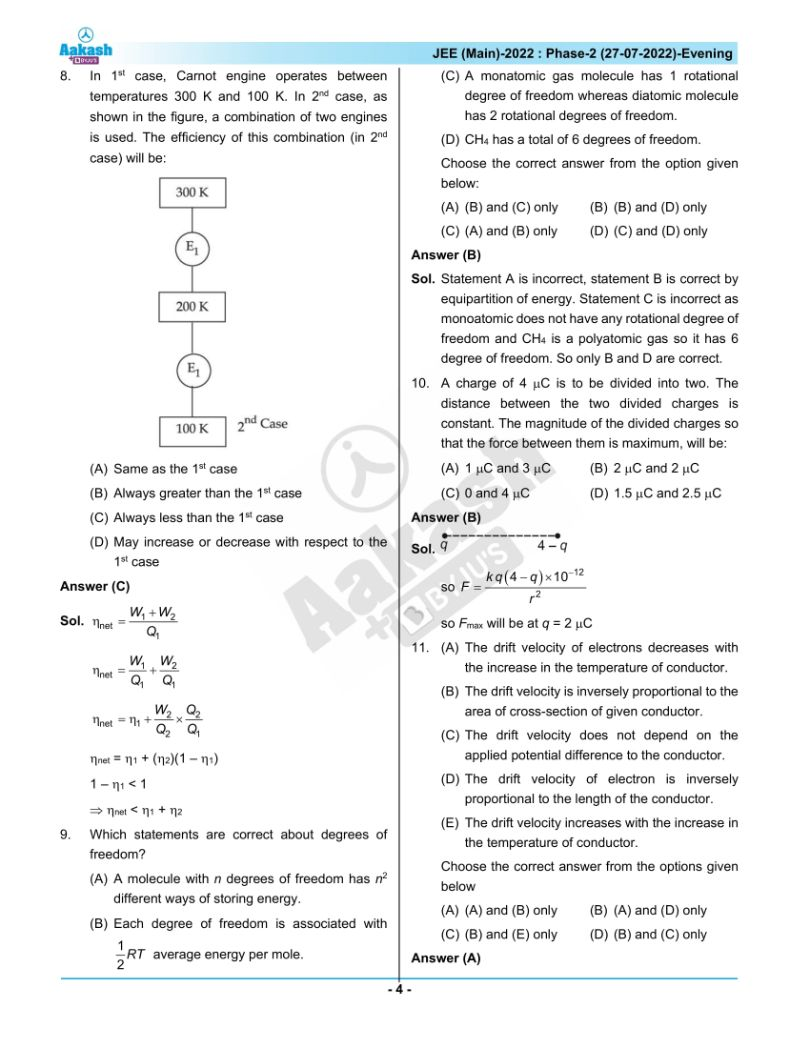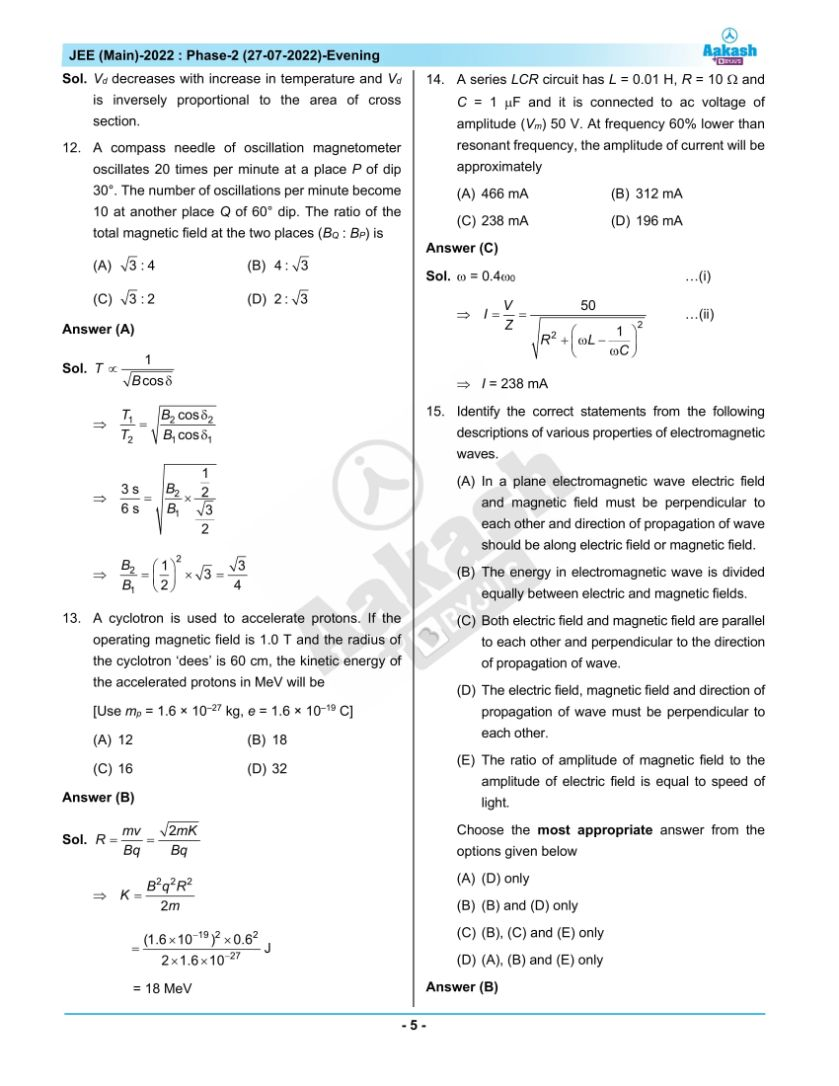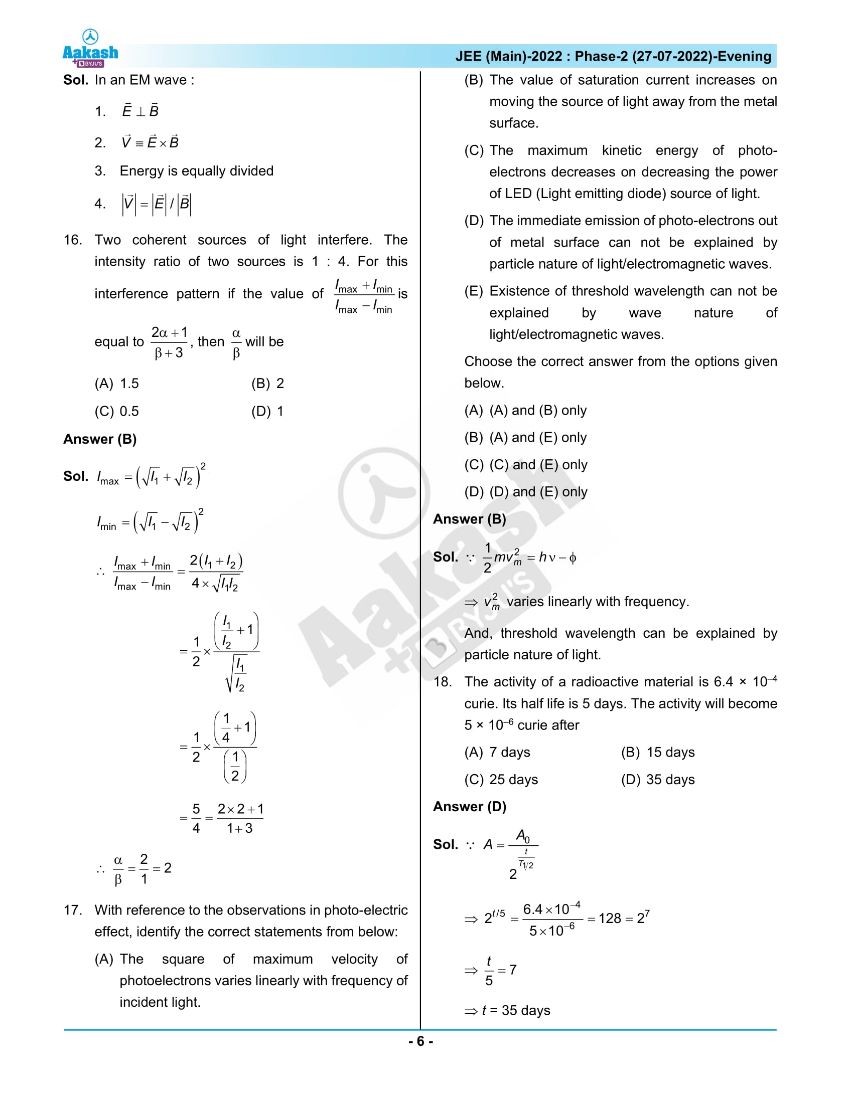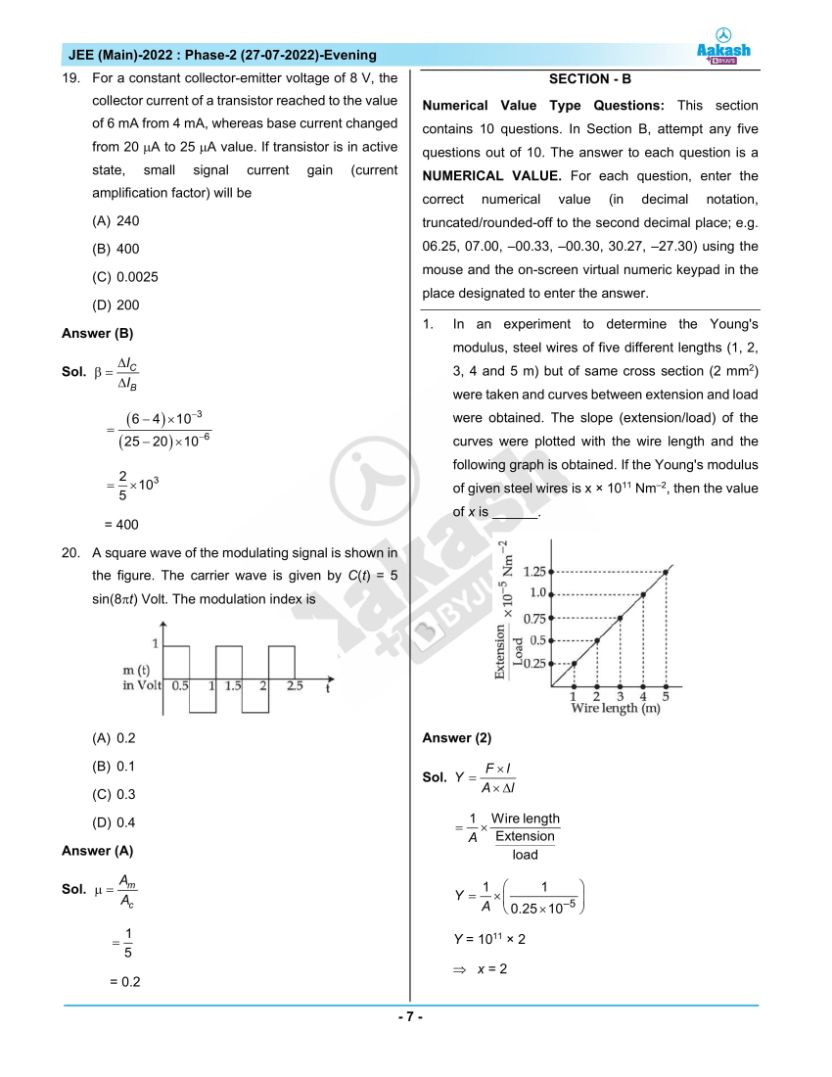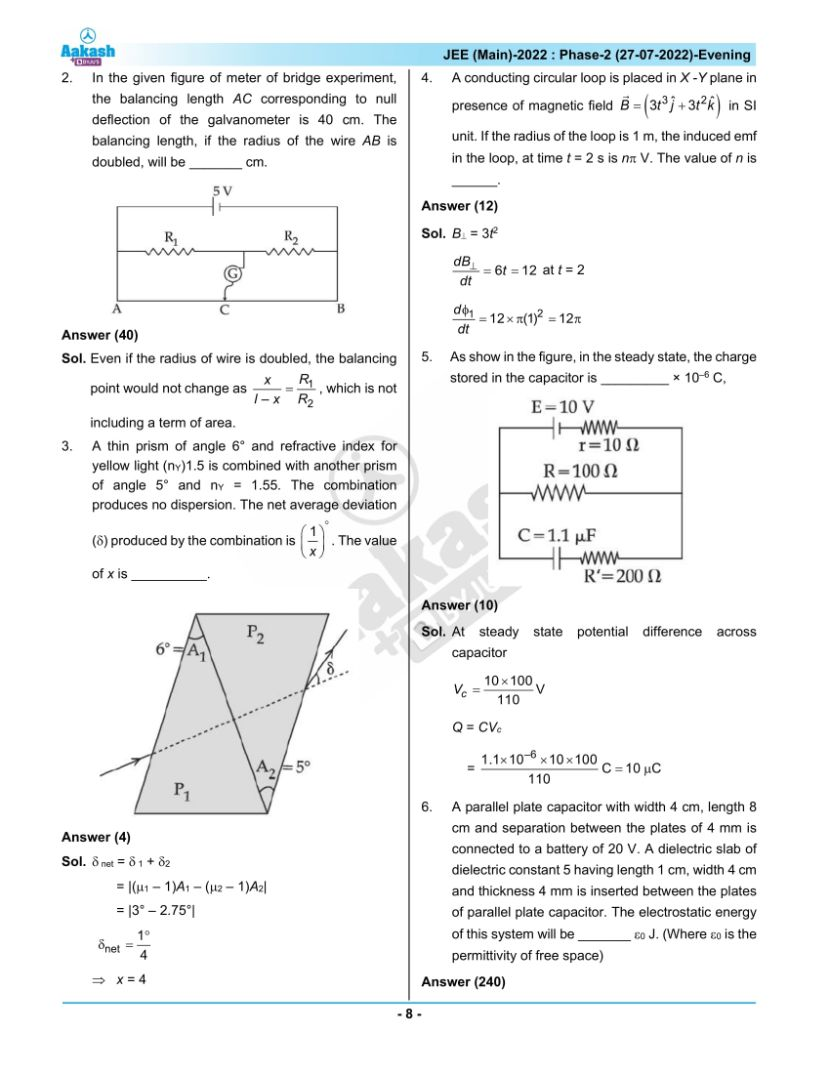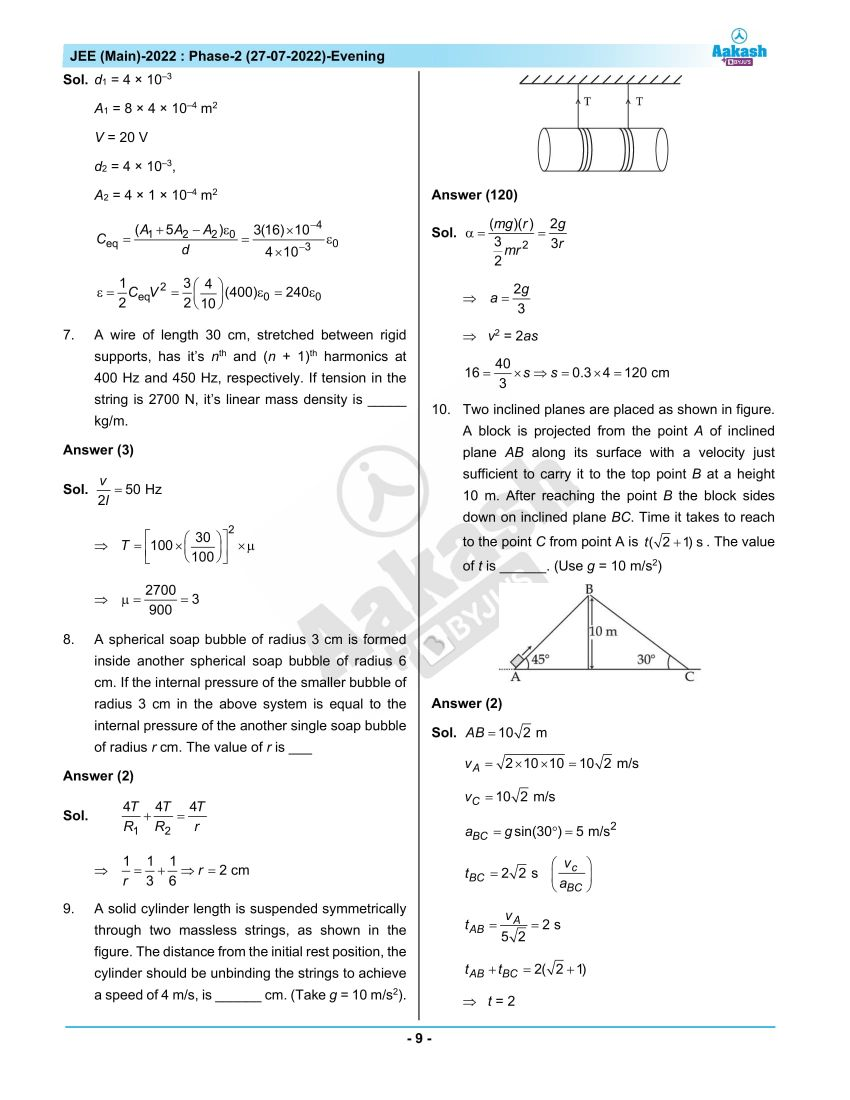## JEE Main 2022 July 27th Shift 2 Paper Analysis